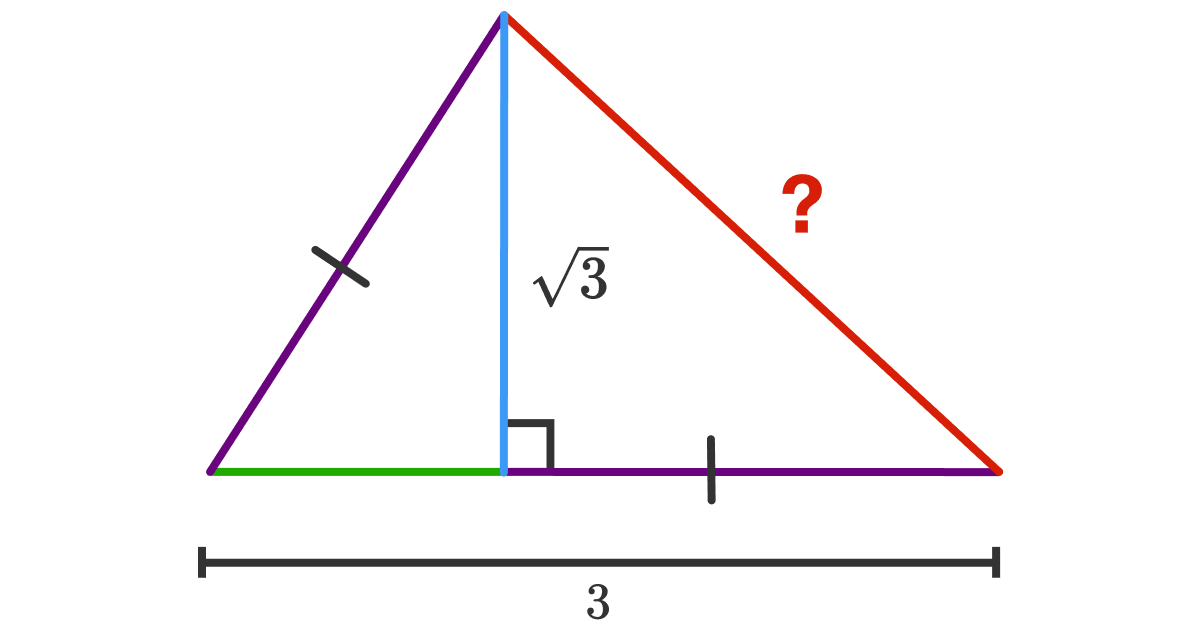# How about rearranging these triangles?

Geometry Level 1The above shows a triangle $ABC$ with base $AB = 3$ and height $CD = \sqrt3$. Point $D$ lies on $AB$ such that $AD= BC$. Find the length of $AC$.

×

Problem Loading...

Note Loading...

Set Loading...